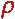Math & Science Home | Proficiency Tests | Mathematical Thinking in Physics | Aeronauts 2000

 CONTENTS Introduction Fermi's Piano Tuner Problem How Old is Old? If the Terrestrial Poles were to Melt... Sunlight Exerts Pressure Falling Eastward What if an Asteroid Hit the Earth Using a Jeep to Estimate the Energy in Gasoline How do Police Radars really work? How "Fast" is the Speed of Light? How Long is a Light Year? How Big is a Trillion? "Seeing" the Earth, Moon, and Sun to Scale Of Stars and Drops of Water If I Were to Build a Model of the Cosmos... A Number Trick Designing a High Altitude Balloon Pressure in the Vicinity of a Lunar Astronaut Space Suit due to Outgassing of Coolant Water Calendar Calculations Telling Time by the Stars - Sidereal Time Fields, an Heuristic Approach The Irrationality ofThe Irrationality ofThe Number (i)i Estimating the Temperature of a Flat Plate in Low Earth Orbit Proving that (p)1/n is Irrational when p is a Prime and n>1 The Transcendentality ofIdeal Gases under Constant Volume, Constant Pressure, Constant Temperature and Adiabatic Conditions Maxwell's Equations: The Vector and Scalar Potentials A Possible Scalar Term Describing Energy Density in the Gravitational Field A Proposed Relativistic, Thermodynamic Four-Vector Motivational Argument for the Expression-eix=cosx+isinx Another Motivational Argument for the Expression-eix=cosx+isinx Calculating the Energy from Sunlight over a 12 hour period Calculating the Energy from Sunlight over actual full day Perfect Numbers-A Case Study Gravitation Inside a Uniform Hollow Sphere Further note on Gravitation Inside a Uniform Hollow Sphere Pythagorean Triples Black Holes and Point Set Topology Additional Notes on Black Holes and Point Set Topology Field Equations and Equations of Motion (General Relativity) The observer in modern physics A Note on the Centrifugal and Coriolis Accelerations as Pseudo Accelerations - PDF File On Expansion of the Universe - PDF File

## What if an Asteroid Hit the Earth?

Problem:
Suppose a cylindrical asteroid 10 km in height x 10 km in diameter impacted the earth at 30,000 mph. Calculate the energy dissipated, in megatons of TNT, and the rate at which it is dissipated, in watts.

Solution:
Let the density of the asteroid,, be five times the density of water, i.e.,= 5000 kg/m3.

Then, the mass of the asteroid is

(5000 kg/m3) x(5000 m)2 x (10,000 m) = 4 x 1015 kg

and the kinetic energy, traveling at 30,000 mph (= 1 x 104 m/sec) is

(1/2) x (4 x 1015 kg) x (1 x 104 m/sec)2 = 2 x 1023 joule.

Now, one megaton of TNT delivers 4.2 x 1015 joule, so that the required energy in megatons of TNT is

5 x 107 megatons

or 50 million megatons! The time for the asteroid to impact the earth is

(10,000 m)/(1 x 104 m/sec) = 1 sec

so that the rate of energy dissipation in watts is

2 x 1023 watts,

or 200 billion trillion watts!!!

Afterthought:
Such an object might have been responsible for causing the extinction of the dinosaurs at the end of the Cretaceous period. A layer of Iridium has been found in the Cretaceous-Tertiary (K-T) boundary, which appears to be world encircling (Alvarez, et. al.). Such a layer might have been deposited after an asteroid impact of great magnitude. In fact, the remains of a 65 million year old impact site have recently been found, providing an excellent candidate for the K-T event. From various geological survey data (originally intended to locate promising sites for oil drilling), the site is in the Yucatan.

Please send suggestions/corrections to:
Web Related: David.Mazza@grc.nasa.gov
Technology Related: Joseph.C.Kolecki@grc.nasa.gov
Responsible NASA Official: Theresa.M.Scott (Acting)